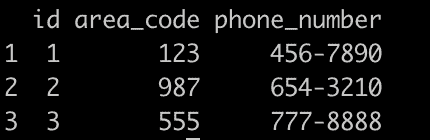# How to Use extract() Function in R

The extract() function from the tidyr package in R is “used to extract multiple values from a single column into multiple columns.”

## Syntax

``extract(data, col, into, regex, remove = TRUE, convert = FALSE)``

## Parameters

1. data: The data frame.
2. col: The column name you want to extract values from.
3. into: A vector of column names you want to create.
4. regex: A regular expression that defines how to extract values.
5. remove: If TRUE, remove the column that you are extracting values from. If FALSE, keep the original column.
6. convert: If TRUE, will automatically convert the extracted values into the appropriate type (numeric, integer, etc.). If FALSE, the new columns will be of type character.

## Example 1: Extracting First Name and Last Name

``````library(tidyr)

# Sample data
df <- data.frame(id = 1:3, full_name = c(
"Alice Brown",
"Bob Smith", "Charlie Johnson"
))

# Use extract() to split the full_name column
df <- df %>%
extract(full_name,
into = c("first_name", "last_name"),
regex = "(\\w+) (\\w+)"
)

print(df)``````

Output## Example 2: Extracting Area Code and Phone Number

Imagine you have phone numbers in the format “(AreaCode) PhoneNumber,” and you want to extract the area code and phone number into separate columns.

``````library(tidyr)

# Sample data
df <- data.frame(id = 1:3, phone = c(
"(123) 456-7890",
"(987) 654-3210", "(555) 777-8888"
))

# Use extract() to split the phone column
df <- df %>%
extract(phone,
into = c("area_code", "phone_number"),
regex = "\\((\\d{3})\\) (\\d{3}-\\d{4})"
)

print(df)
``````

OutputThat’s it!

## Related posts

separate() function in R